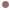Autres Articles de :
Discrete maps

# The Logistic Map

Christophe LETELLIER
11/01/2008Historical accountPierre François de Verhulst

In 1845, the discrete map was introduced in its continuous form by Pierre-François de Verhulst (1804-1849) . It is the first order differential equationwhere N is the number of individuals,is the maximum growth rate, andis the biotic capacity. Dividing byand using, the most often form of the logistic equation is obtained, that is,It was investigated in its discrete formby Pekka Juhana Myrberg (1892–1976) in the early 1960s  : in particular, Myrberg identified the period-doubling cascade and the corresponding accumulation point. In fact, Myrberg did not investigate one map, but a family of quadratic one-dimensional continuous mapson the interval I. For all maps, the accumulation point is provided bywhereis the parameter value at which the orbit of period 20=1 occurs. In 1964, Andrei Sharkovskii obtained a theorem providing the order with which period-p orbits appear for the first time when the bifurcation parameter is varied .

In 1973, Metropolis, Stein & Stein investigated quadratic maps - the logistic map being a simple particular example - and found that the order in which all limit cycles were observed was universal’’, that is, was not dependent on the specific algebraic form of the map considered . An important aspect of their work is that they used symbolic dynamics. In 1974, using numerical simulations, Robert May rediscovered the period-doubling cascades and showed that chaotic regimes occur after the accumulation point . May then used the logistic map to show that a simple map can induce very complicated behaviors . In 1975, Igor Gumowski and Christian Mira showed that the sequences of period-doubling cascades was fractal in the sense that the whole bifurcation diagram can be recovered in a part of it : they called this the box-within-the box structure . In 1977, John Guckenheimer provided a formal introduction to the `universal’’ sequence of bifurcations which take place with respect to the bifurcation parameter. The family of maps considered have the following properties :is a smooth function and has a single critical point (quadratic maps are thus included, but unimodal maps is a more general designation). Guckenheimer provided an algorithm for computing the order of the period-p of the stable limit cycle observed while varying the bifurcation parameter.

In 1978, Pierre Coullet and Charles Tresser  and Mitchell Feigenbaum  independently investigated the renormalization properties of continuous unimodal maps and found that there is a scaling law on the parameters.

The logistic map is thus a very convenient example to start to investigate the period-doubling cascade as a route to chaos. It should be keep in mind, that it also serve as a model for the first-return map to a Poincaré section of any set of differential equations which produces a period-doubling cascade as a route to chaos.Dynamical analysis

The logistic map presents a period-doubling cascade as a route to chaos as shown by a bifurcation diagram (Fig. 1). The Logistic map has two period-1 points : x0=0 and. The point x0 is stable on the range. The second period-1 point is stable on the range. These two period-1 points exchange their stability through a transcritical bifurcation. Then the point x0 becomes unstable through a period-doubling birfucation. This first period-doubling bifurcation is followed by a cascade of period-doubling bifurcations up to the accumulation point. Then a first chaotic regime is observed. Many other period-doubling cascades can be found in this bifurcation diagram. Let us note the cascade emerging from the stable period-3 limit cycle appearing at.Fig. 1. Bifurcation diagram of the logistic map.

When a blow-up is made within the period-3 window, a picture equivalent to the full bifurcation diagram is recovered (Fig. 2). This is a simple illustration of the nature (the box-within-the box structure) of the bifurcation diagram. The order with which all the limit cycles when parameteris increased can be predicted by the so-called unimodal order, built from the symbolic dynamics based on the partitionFig. 2. Period-3 window. A period-doubling cascade is observed from the stable period-3 limit cycle.

 P. F. de Verhulst, Recherches mathématiques sur la loi d’accroissement de la population, Nouveaux Mémoires de l’Académie Royale des Sciences et Belles-Lettres de Bruxelles, 18, 1-42, 1845.

 P. J. Myrberg, Sur l’itération des polynômes réels quadratiques, Journal de Mathématiques pures et appliquées 9 (41), 339-351, 1962.

 A. N. Sharkovskii, Coexistence of cycles of a continuous map of the line into itself, Ukrain. Mat. Zh. , 16, 61–71, 1964.

 N. Metropolis, M. L. Stein & P. R. Stein, On finite limit sets for transformations on the unit interval, Journal of Combinatorial Theory A , 15, 25–44, 1973.

 R. May, Biological populations with nonoverlapping Generations : stable points, stable cycles, and chaos, Science, 186, 645-647, 1974.

 R. M. May, Simple mathematical models with very complicated dynamics, Nature, 261, 459 - 467, 1976.

 I. Gumowski & C. Mira, Accumulations de bifurcations dans une récurrence, Comptes-Rendus de l’Académie des Sciences, 281, 45-48, 1975.

 J. Guckenheimer, On the bifurcation of maps of the interval, Inventiones Mathematicae, 39, 165-178, 1977.

 P. Coullet & C. Tresser, Itérations d’endomorphismes et groupe de rénormalisation, Journal de Physique, C5, 25–28, 1978.

 M. Feigenbaum, Quantitative universality for a class of non-linear transformations, Journal of Statistical Physics, 19, 25–52, 1978.